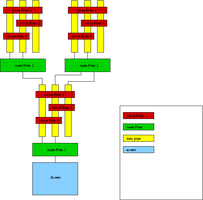## MorbitMorbit is an architecture for efficiently building arbitrary function tree's. I use it to generate most of the fractals that I play with and its an ongoing project.

The Morbit architecture allows me to connect together objects that I call filters. A filter can be as simple as taking the inverse of a number, or as complex as generating the orbits of itterated non-linear equations. These filters are divided into two classes.

An InlineFilter is the simpler of the two filter classes. An InlineFilter will use exactly as much data as it generates. An example of an inline filter is a filter that takes complex numbers and adds noise with some distribution. Or a filter that takes a vector and rotates it in space.

The NodeFilter is the other type of filter. These filters are not restricted to generating the same number of data entries as they use. They are more complex in implimentation, but provide a greater flexability. An example of a NodeFilter would be a filter that takes Complex numbers and uses them as the initial value or a constant in an itterated system. This is very common in fractals. The filter could output many points from the orbit that was seeded by a single input.

Here are a couple examples of what the system can generate in its current form. These use the InverseItterationMethod to compute a boundary of a julia set and then feed those points to be the initial values for the forward itteration of a different julia set.

These are MPEG animations that vary the distribution of initial points that are used to seed the orbits of the famous z(n+1) = z(n)^2 + c map.